# Square-1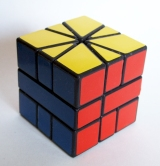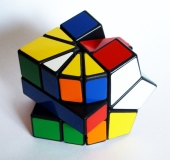The Square-1 (previously called as Cube 21 and Back to Square One) is a shape-shifting three-layered twisty puzzle. Its solution is very unique because the kite-shaped corners and the triangular edges are indistinguishable to the puzzle's inner mechanism, meaning that corners can be swapped with edges and therefore it's possible to have 10 pieces in the upper layer while only 6 in the bottom.

The puzzle was invented in 1990 by Karel Hršel and Vojtech Kopský. It's an official WCA competition event, the fastest solution being held by Martin Vædele Egdal (4.59 seconds).Variations: Super Square-1, Square-2, Two Layered and Four Layered versions

## How to solve the Square-1

The main idea of the solution is the same as the Rubik's Cube method: We divide the puzzle into layers and solve them one by one, not messing up the pieces already fixed. This puzzle has a completely different mechanism, so we have to introduce new notations and algorithms.

### Notation

The top and bottom layers of a Square-1 are made of thin triangle edges and thick kite-shaped corner pieces. An edge piece is 30o (1 step), while a thick piece is 60o (2 steps) wide.In the algorithms we specify how many steps to turn the top and bottom layers between the slice moves.

/ - a slice is like a 180o R rotation on the Rubik's Cube (image). Square-1 scramblers usually neglect the slice move.
(1, 0) / - rotate the top layer 30o clockwise and slice
(0, 3) / - rotate the bottom layer 90o and slice. Equivalent to the D move on the Rubik's Cube.
/ (0, -1) / - start with a slice, rotate bottom layer 30o counterclockwise then slice again
(2, -1) / - rotate the top layer 2 steps, the bottom anticlockwise 1 step and do a slice

### 1. Bring the puzzle to a square shape

The puzzle is much easier to work with when it is in the shape of a cube. Try to bring the Square-1 to a cube shape in the first step. This is not a complicated stage because there are no solved pieces you can mess up. Use this step to familiarize with its operation.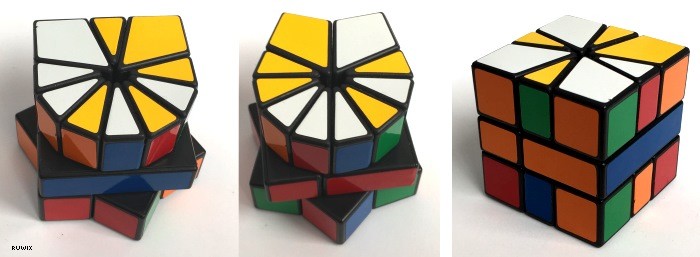Try to group the small pieces together to form a cube with the method below

The two examples below describe how to make a cube from two easy-to-reach positions, when the thick pieces are collected in the bottom and the narrow pieces are grouped together on the top, as much as possible.

Case 1: If every small piece is grouped in the top layer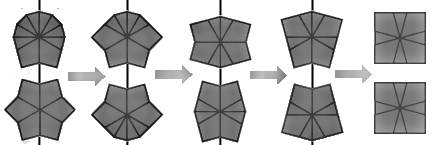Case 2: If there's a lonely small piece in the top layerTo bring the cube to a square shape first you need to collect the tiny pieces next to each other or leave maximum one lonely piece between two thick corners. This is not that hard to accomplish, it can be done intuitively. When this is done follow the steps on the pictures above. The black vertical line marks where to make the slice

### Wrong middle layer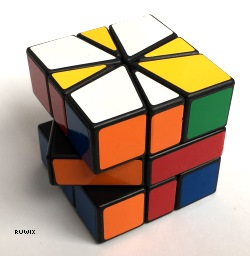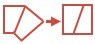If the middle layer is not square, do the following: (0, -1) / (6, 0) / (6, 0) / (0, 1)

Now that the shape of the puzzle is a cube, we can easily handle the pieces.

### 2. Top (yellow) corners

First bring all corner pieces to the corresponding layer: Hold the cube with the red side facing you and the green on the right. The yellow has to come to the top and the white to the bottom. This step is not so complicated, it can be done intuitively, if not, here's a little help to swith two pieces in the top and bottom layers: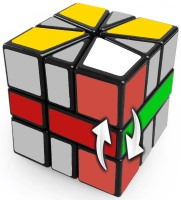(0, -4) / (0, 3) / (0, 1)

When every corner is in the correct layer, bring the yellow corners to their final position, swithching two pieces in the top right side: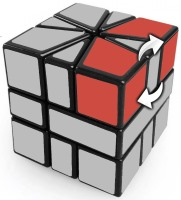(1, 0) / (0, -3) / (0, 3) / (0, -3) / (0, -3) / (0, 6) / (-1, 0)

### 3. Edges to their layers

Yellow edges to the top, white edges to the bottom layer. To swap two pieces move them to the top right and bottom right of the cube then do the algorithm.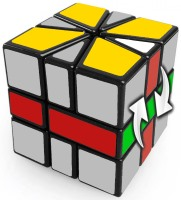(1, 0) / (0, -3) / (0, -3) / (-1, -1) / (1, 4) / (0, 3) / (-1, 0)

Repeat this until every edge gets to its layer. It doesn't matter if they're not on the exact final spot. At the end of this step you should see the white and yellow faces solved.

### 4. Swap corners

In the second step we sent every top-layer corners to their final position. Now do the bottom corners. Use the trick below to swap the two front-facing corners in the bottom layer./ (3, -3) / (0, 3) / (-3, 0) / (3, 0) / (-3, 0) /

### 5. Permute the edges

At this point every edge should be at its correct layer, we just have to put them to their final position.

Switch two pieces on the top and two in the bottom layer at the same time. The algorithm below switches the right-top with the back-top edge and the right-bottom with the back-bottom edge.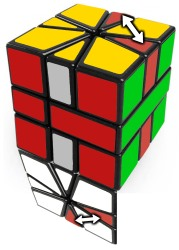(0, 2) / (0, -3) / (1, 1) / (-1, 2) / (0, -2)

In most cases your Square One puzzle should be solved at the end of this step. If there are two edges that need to be fixed then you have a parity.

### 6. Parity

If there are only two edges left to finish the cube then you've got parity. Use this long algorithm to switch two edges on the top then go back to point 5.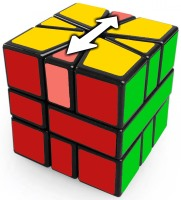/ (3,3) / (1,0) / (-2,-2) / (2,0) / (2,2) / (-1,0) / (-3,-3) / (-2,0) / (3,3) / (3,0) / (-1,-1) / (-3,0) / (1,1) / (-4,-3)